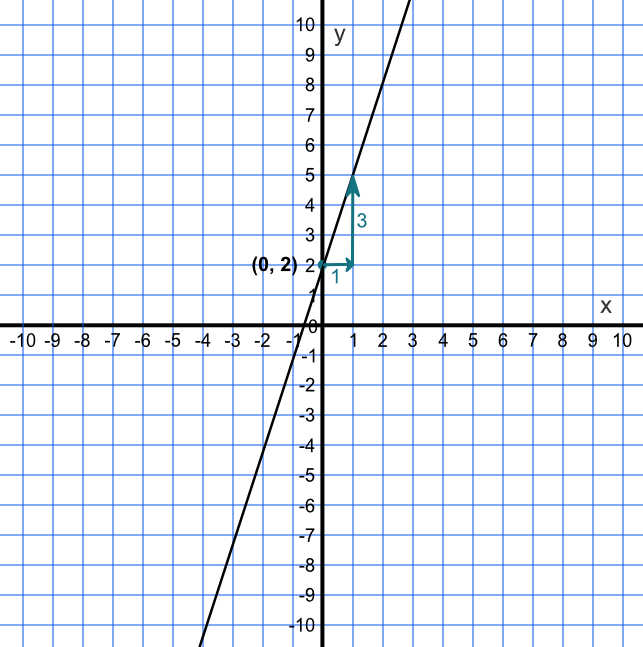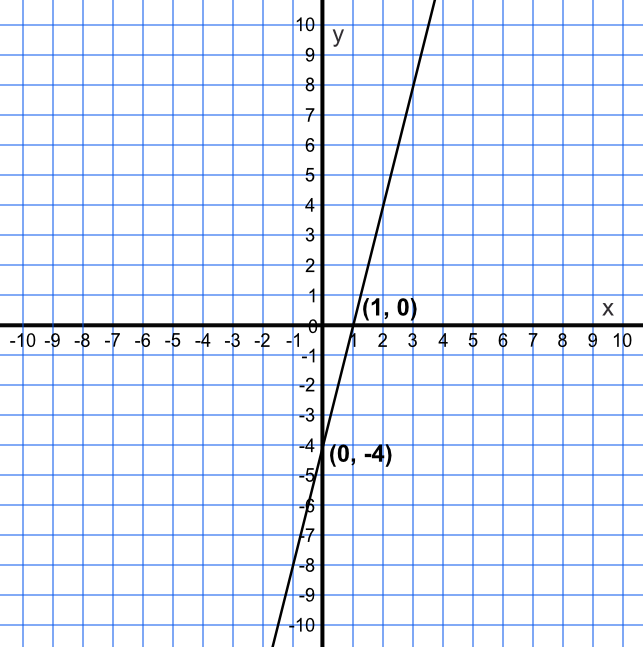Gradients and Intercepts of Linear Functions

## Gradients and Intercepts of Linear Functions

A Linear Function represents a constant rate of change. When plotted on a graph it will be a straight line.

A graph may be plotted from an equation y = mx + c by plotting the intercept at (0, c), and then drawing the gradient m, although it is normally easier to generate the points in a table and plot the graph.

The graph of a line can be used to find the equation by working out the gradient frac(up)(along) which gives the m value, while the intercept on the y-axis gives the c value.

## Example 1

Draw a graph for the equation y = 3x + 2

Either plot a table for values of x and y or,

Using y = mx + c:

c is the intercept y = 2 when x = 0 and

m is the gradient = 3 (3 up, 1 along)## Example 2

Without drawing a graph, determine the coordinates for the intercepts on the x- and y-axes for y = 4x - 4.

When x = 0, then y = 4 xx 0 - 4 therefore y = -4. Coordinate is (0, -4)

When y = 0, then 0 =4x - 4 therefore x = 1. Coordinate is (1, 0)Answer: (0, -4) and (4, 0)